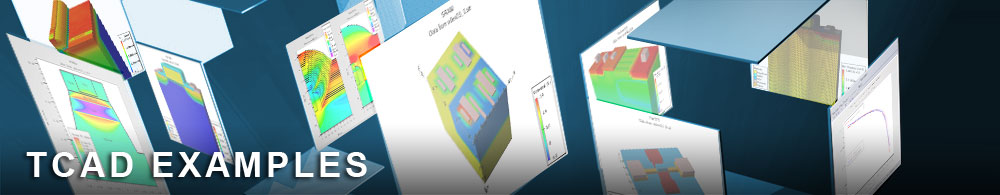Modelling for Deep Submicron - Process to Device

## soiex08.in : Modelling for Deep Submicron - Process to Device

Requires: SSuprem 4/S-Pisces/Giga
Minimum Versions: Athena 5.22.3.R, Atlas 5.28.1.R

This example uses process simulation to construct a SOI MOSFET that has full dielectric isolation. Device simulations are then performed that use Non-isothermal Energy Balance (NEB) calculations to obtain Ids/Vds characteristics. It shows:

• SOI structure formation using SSuprem 4 process simulation
• Partially depleted SOI design
• Selection of energy balance and lattice heating models in Atlas
• Selection of numerical methods for coupled solution technique
• Id/Vds simulation for Vgs=1.0, 3.0 and 5.0 V

A silicon-oxide-silicon structure was formed in SSuprem 4 by use of the region statement of Athena. This structure was then subjected to a standard bulk MOSFET processing sequence, which has been described in mos1ex01.in. The structure in this example has been designed so that the SOI thickness is greater than the depth of the source and drain junctions. This ensures that the device will operate in a partially depleted mode of operation during later device simulations.

One major modification made to the original processing sequence was to introduce a LOCOS isolation step. The result of this step was the creation of a fully oxide isolated island of silicon. The COMPRESS model for oxidation has been used in this example but more complex and accurate models may be used to study birds beak effects in SOI MOSFETs, as described in the Athena/SSuprem 4 section.

The process simulation structure will be passed into Atlas automatically using DeckBuild's auto-interface. This auto-interface therefore allows global optimization from process simulation to device simulation to SPICE model parameter extraction.

The contact statement is used in Atlas to define the workfunction of the gate electrode. The interface command is used to define the the fixed charge at the oxide/silicon interfaces. The models statement defines a set of physical models for the simulation. CVT and SRH are standard models for MOSFET simulation. The energy balance equation for electrons is specified by hcte.el parameter and the lattice heat flow equation is solved with Giga by setting the parameter lat.temp . A thermal contact must also be defined when solving the heat flow equation with Giga and is defined using the thermcontact statement. In this example this thermal electrode is defined to be the same as contact number #4 which is the substrate electrode.

The numerical algorithm chosen for this system of equations is the newton approach defined on the method statement. This statement is also used to define the semiconductor equations. carr=2 will solve for potential and the hole and electron continuity equations. The trap parameter allows the bias step to be reduced in cases of non-convergence for a total of maxtrap times.

The solution begins by applying zero volts to all contacts and only solving for potential. A small voltage is then applied to the drain contact. This is normally advised in the case of SOI simulations due to the presence of a floating region of potential. As described in previous SOI examples, this floating region of potential can cause great numerical difficulties. However by solving for 5 equations, which couples the equations very tightly, and applying only small voltage steps the convergence in this example is excellent.

The gate voltage is ramped to 1, 2 and 3 V and a solution saved at each of these bias conditions. Each solution is then used as the initial starting point for simulating the Ids/Vds characteristics by using the load command. A logfile is then opened to store the terminal characteristics and a solve statement applies the drain bias sweep. This procedure is then repeated for each gate voltage.

To load and run this example, select the Load button in DeckBuild > Examples. This will copy the input file and any support files to your current working directory. Select the Run button in DeckBuild to execute the example.